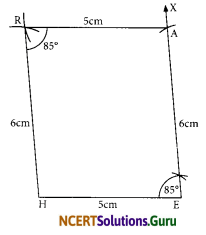# NCERT Solutions for Class 8 Maths Chapter 4 Practical Geometry Ex 4.3

These NCERT Solutions for Class 8 Maths Chapter 4 Practical Geometry Ex 4.3 Questions and Answers are prepared by our highly skilled subject experts.

## NCERT Solutions for Class 8 Maths Chapter 4 Practical Geometry Exercise 4.3

Question 1.
MO = 6 cm
OR = 4.5 cm
∠M = 60°
∠O = 105°
∠R = 105°

PL = 4 cm
∠A = 6.5 cm
∠P = 90°
∠A = 110°
∠N = 85°(iii) Parallelogram HEAR
HE = 5 cm
EA = 6 cm
∠R = 85°

(iv) Rectangle OKAY
OK = 7 cm
KA = 5 cm
Solution:
(i)Steps of construction:
I. Draw a line segment MO = 6 cm.
II. At M, draw $$\overrightarrow{\mathrm{MX}}$$, such that ∠OMX = 60°
III. At O, draw $$\overrightarrow{\mathrm{OY}}$$, such that ∠MOY = 105°
IV. From $$\overrightarrow{\mathrm{OY}}$$, cut off OR = 4.5 cm.
V. At R, draw RZ, such that ∠ORZ = 105°. Let $$\overrightarrow{\mathrm{RZ}}$$ intersects $$\overrightarrow{\mathrm{MX}}$$ at E. Thus, MORE is the required quadrilateral.

(ii)Steps of construction:
I. Draw a line segment PL = 4 cm
II. At L, draw $$\overrightarrow{\mathrm{LX}}$$ such that ∠PLX = 75°
III. From $$\overrightarrow{\mathrm{LX}}$$, cut off LA = 6.5 cm
IV. At A, draw $$\overrightarrow{\mathrm{AY}}$$ such that ∠LAY = 110°
V. At P, draw $$\overrightarrow{\mathrm{PZ}}$$ such that ∠LPZ = 90°. Let $$\overrightarrow{\mathrm{PZ}}$$ and $$\overrightarrow{\mathrm{AY}}$$ intersect at N. Thus, PLAN is required quadrilateral.(iii)Steps of Construction:
I. Draw a line segment $$\overrightarrow{\mathrm{HE}}$$ = 5 cm
II. At E, draw $$\overrightarrow{\mathrm{EX}}$$ such that ∠HEA = 85°
III. From $$\overrightarrow{\mathrm{EX}}$$, cut-off EA = 6 cm.
IV. With centre at A and radius = 5 cm, draw an arc.
V. With centre at H and radius = 6 cm, draw an arc such that it intersects the previous arc at R.
VI. Join RA and RH. Thus, HEAR is the required parallelogram.

(iv)Steps of Construction:
I. Draw a line segment OK = 7 cm.
II. At O, draw $$\overrightarrow{\mathrm{OP}}$$ such that ∠KOP = 90°.
III. From $$\overrightarrow{\mathrm{OP}}$$, cut-off $$\overrightarrow{\mathrm{OY}}$$ = 5 cm.
IV. At K, draw $$\overrightarrow{\mathrm{KQ}}$$ such that ∠OKQ = 90°
V. From $$\overrightarrow{\mathrm{KQ}}$$, cut-off $$\overrightarrow{\mathrm{KA}}$$ = 5 cm.
VI. Join AY. Thus, OKAY is the required rectangle.

error: Content is protected !!